# Turan, Paul

## Turán's problem for hypergraphs ★★

Author(s): Turan

Conjecture   Every simple-uniform hypergraph on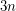vertices which contains no complete-uniform hypergraph on four vertices has at mosthyperedges.
Conjecture   Every simple-uniform hypergraph on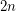vertices which contains no complete-uniform hypergraph on five vertices has at most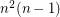hyperedges.

Keywords:

## The Erdos-Turan conjecture on additive bases ★★★★

Author(s): Erdos; Turan

Let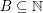. The representation function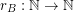foris given by the rule. We callan additive basis if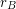is never.

Conjecture   Ifis an additive basis, thenis unbounded.

Keywords: additive basis; representation function

## The Crossing Number of the Complete Bipartite Graph ★★★

Author(s): Turan

The crossing number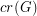ofis the minimum number of crossings in all drawings ofin the plane.

ConjectureKeywords: complete bipartite graph; crossing number